# Iranian Navy QPSK Modem

Iranian Navy QPSK Modem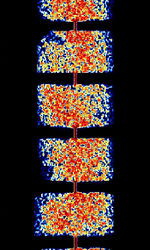Frequencies 8.475 MHz,8.046 MHz,10.724 MHz,17.3822 MHz
Frequency Range 8.046 MHz - 17.3822 MHz
Mode USB
Modulation PSK
ACF
Emission Designator
Bandwidth 700 Hz,300 Hz,620 Hz,1.25 kHz,2.52 kHz,1.42 kHz,2.85 kHz
Location Iran
Short Description Iranian Navy QPSK Modem is a QPSK mode used by the Iranian Navy. It has gone through several versions. The current version (2015) is V2 and supports speeds of 468 Bd, 936 Bd, and 1872 Bd.
I/Q Raw Recording
Audio Sample

## Description

Iranian Navy QPSKQuadrature Phase-Shift Keying (2 bits per symbol) Modem is a QPSKQuadrature Phase-Shift Keying (2 bits per symbol) mode used by the Iranian Navy. It has gone through several versions. The current version (2015) is V2 and supports speeds of 468 BdBaud (unit symbol Bd) is the unit for symbol rate or modulation rate in symbols per second., 936 BdBaud (unit symbol Bd) is the unit for symbol rate or modulation rate in symbols per second., and 1872 BdBaud (unit symbol Bd) is the unit for symbol rate or modulation rate in symbols per second..

## Characteristics

### Original QPSKQuadrature Phase-Shift Keying (2 bits per symbol) 207 BdBaud (unit symbol Bd) is the unit for symbol rate or modulation rate in symbols per second. Modem

The original Iranian Navy QPSKQuadrature Phase-Shift Keying (2 bits per symbol) Modem only supported one baudrate, 207 BdBaud (unit symbol Bd) is the unit for symbol rate or modulation rate in symbols per second.. The data is transmitted in packets, and each transmission starts with an unmodulated pre-carrier of 2000 HzHertz (Hz), unit of frequency, defined as one cycle per second (1 Hz).. 100 msmilliseconds (.001 of a second) before data transmission, the carrier has a phase jump of 180 degrees. At the end of each transmission two tones are sent at 1000Hz, first one 100ms long and the second one 110 msmilliseconds (.001 of a second) long right after the first. Total length of this two-tone end of message transmission is ~250 msmilliseconds (.001 of a second).

V1 Adaptive Modem is a further development of the original QPSKQuadrature Phase-Shift Keying (2 bits per symbol) modem. V1 supports four different speeds: 207 BdBaud (unit symbol Bd) is the unit for symbol rate or modulation rate in symbols per second., 414 BdBaud (unit symbol Bd) is the unit for symbol rate or modulation rate in symbols per second., 828 BdBaud (unit symbol Bd) is the unit for symbol rate or modulation rate in symbols per second., and 1656 BdBaud (unit symbol Bd) is the unit for symbol rate or modulation rate in symbols per second. and all are QPSKQuadrature Phase-Shift Keying (2 bits per symbol) modulated. Like the original QPSKQuadrature Phase-Shift Keying (2 bits per symbol) modem, each packet is sent with a pre-carrier burst.

• 207 BdBaud (unit symbol Bd) is the unit for symbol rate or modulation rate in symbols per second. (414 bpsBits per second (bps)), 300 HzHertz (Hz), unit of frequency, defined as one cycle per second (1 Hz). Bandwidth
• 414 BdBaud (unit symbol Bd) is the unit for symbol rate or modulation rate in symbols per second. (828 bpsBits per second (bps)), ~620 HzHertz (Hz), unit of frequency, defined as one cycle per second (1 Hz). Bandwidth (approx)
• 828 BdBaud (unit symbol Bd) is the unit for symbol rate or modulation rate in symbols per second. (1656 bpsBits per second (bps)), ~1250 HzHertz (Hz), unit of frequency, defined as one cycle per second (1 Hz). Bandwidth (approx)
• 1656 BdBaud (unit symbol Bd) is the unit for symbol rate or modulation rate in symbols per second. (3312 bpsBits per second (bps)), ~2520 HzHertz (Hz), unit of frequency, defined as one cycle per second (1 Hz). Bandwidth (approx)

V2 is an upgrade of the V1 Adaptive modem, replacing V1 and using different speeds of 468 BdBaud (unit symbol Bd) is the unit for symbol rate or modulation rate in symbols per second., 936 BdBaud (unit symbol Bd) is the unit for symbol rate or modulation rate in symbols per second., and 1872 BdBaud (unit symbol Bd) is the unit for symbol rate or modulation rate in symbols per second.. All use QPSKQuadrature Phase-Shift Keying (2 bits per symbol) modulation and like the original QPSKQuadrature Phase-Shift Keying (2 bits per symbol) modem, each packet is sent with a pre-carrier burst.

• 468 BdBaud (unit symbol Bd) is the unit for symbol rate or modulation rate in symbols per second. (936 bpsBits per second (bps)), 700 HzHertz (Hz), unit of frequency, defined as one cycle per second (1 Hz). Bandwidth: 1500 msmilliseconds (.001 of a second) long packets are sent with a 430 msmilliseconds (.001 of a second) long pre-carrier burst.

• 936 BdBaud (unit symbol Bd) is the unit for symbol rate or modulation rate in symbols per second. (1872 bpsBits per second (bps)), ~1420 HzHertz (Hz), unit of frequency, defined as one cycle per second (1 Hz). Bandwidth (approx)
• 1872 BdBaud (unit symbol Bd) is the unit for symbol rate or modulation rate in symbols per second. (3744 bpsBits per second (bps)), ~2850 HzHertz (Hz), unit of frequency, defined as one cycle per second (1 Hz). Bandwidth (approx)

## Samples

Original
QPSKQuadrature Phase-Shift Keying (2 bits per symbol) 207 BdBaud (unit symbol Bd) is the unit for symbol rate or modulation rate in symbols per second.
V2 QPSKQuadrature Phase-Shift Keying (2 bits per symbol)
468 BdBaud (unit symbol Bd) is the unit for symbol rate or modulation rate in symbols per second.

 Analysis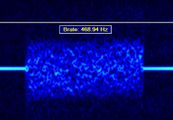PSKPhase-Shift Keying 468 BdBaud (unit symbol Bd) is the unit for symbol rate or modulation rate in symbols per second. analysis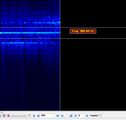PSKPhase-Shift Keying 468 BdBaud (unit symbol Bd) is the unit for symbol rate or modulation rate in symbols per second. analysis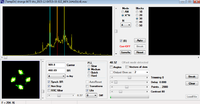PSKPhase-Shift Keying 468 BdBaud (unit symbol Bd) is the unit for symbol rate or modulation rate in symbols per second. analysis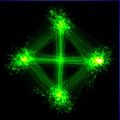PSKPhase-Shift Keying 468 BdBaud (unit symbol Bd) is the unit for symbol rate or modulation rate in symbols per second. analysis
 Images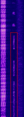Waterfall Image of PSKPhase-Shift Keying 468 BdBaud (unit symbol Bd) is the unit for symbol rate or modulation rate in symbols per second. Signal from WebSDR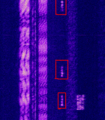The bursts of this PSKPhase-Shift Keying 468 BdBaud (unit symbol Bd) is the unit for symbol rate or modulation rate in symbols per second. signal seen